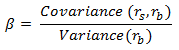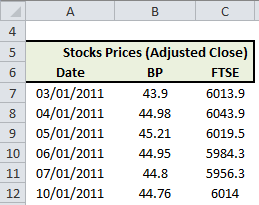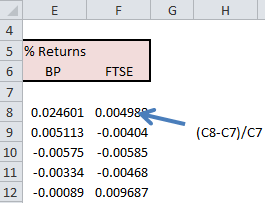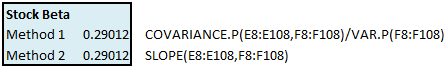# Calculate Stock Beta with Excel

This Excel spreadsheet calculates the beta of a stock, a widely used risk management tool that describes the risk of a single stock with respect to the risk of the overall market. Beta is defined by the following equationwhere rs is the return on the stock and rb is the return on a benchmark index.

## What Does Beta Mean for Investors?

A stock with a beta of

• zero indicates no correlation with the chosen benchmark (e.g. cash or treasury bills)
• one indicates a stock has the same volatility as the market
• more than one indicates a stock that’s more volatile than its benchmark
• less than one is less volatile than its benchmark
• 1.3 is 30% more volatile than its benchmark

Tobacco and utility (e.g. gas & electricity) companies, traditionally regarded as stable and dividend-paying, have low betas while technology companies have higher values. Stocks with a beta of above one should have returns greater than the benchmark index, otherwise it is not regarded as a good investment.

If the benchmark returns 5%, then a stock with a beta of 1.5 should return 1.5 times 5% = 7.5% or more. If not, other investments should be considered instead.

Investments with negative betas have counter cyclical volatility with respect to their benchmark.  In an economy that’s decreasing, gold, bankruptcy advisory firms and companies involved in continuing education often have a beta of less than zero.

There are, however, significant dissadvantages to beta.

1. It’s calculated from historical data (and hence does not capture future changes in the market), and of course depends on the chosen time period.
2. Beta does not discrimnate between upwards volatility and downwards volatility.
3. It assumes that volatility is described by a normal distribution – this isn’t always the case

In summary, beta should only be used in conjunction with other tools when you decide what to invest in.

You can get a list of stocks ordered by their beta at Yahoo Finance, but we’ll now describe how you can calculate it in Excel.

## Historical Stock Returns

We first need a stock and a nominated benchmark index – I’ll pick BP and the FTSE.  Then we need historical stock prices for both.  I used this spreadsheet for downloading historical stock data from Yahoo to get daily closing prices for BP and the FTSE index between 3rd January 2011 and 1st July 2011.Then we simply calculate the fractional daily returns, as described in the picture below.Note that cell range E8:E108 contains the stock returns and the cell range F8:F108 contains the index returns

## Beta Calculation

There are two ways of calculating beta with Excel – the first uses the variance and covariance functions, while the second uses the slope function.The corresponding formulae are given below.

1. `=COVARIANCE.P(E8:E108,F8:F108)/VAR.P(F8:F108)`
2. `=SLOPE(E8:E108,F8:F108)`You could also calculate beta simply by plotting the benchmark returns against the stock returns, and adding a linear trendline. Beta is then simply the slope of the trendline.

Here we see that BP has a beta of 0.29. This is low, and implies that BP’s stock price does not vary significantly when the FTSE swings up and down.### 10 thoughts on “Calculate Stock Beta with Excel”

2. the formula used in method 1 is not working in the excel. In the excel formula COVAR is working and not not COVARIANCE.P. The same thing in the case of Variance. Therefor the result differs. Kindly let me know how do this work.
my another question is what exact formula is excel using so that we can use it without excel manually.

• Both methods work for me. I’ve tested the spreadsheet on Excel 2010 and Excel 2013. COVARIANCE.P was introduced with Excel 2010, so it won’t work if you’re using Excel 2007.

If you want the formulas that Excel uses to calculate variance and covariance, then Wikipedia is a great reference:
http://en.wikipedia.org/wiki/Covariance
http://en.wikipedia.org/wiki/Variance
They can be implemented with basic Excel functions like sum() etc

• Yo can use the Slope command to get he beta of the sock